|

# 跨界进入全屋定制 布兰莎品牌战略发布会惊艳羊城

板材翘楚大王椰集团20亿重金打造的布兰莎全屋定制，重磅出击全屋定制领域，将带来哪些惊喜？3月31日下午,"起步与世界同步——2017布兰莎品牌战略发布会"在广州广交会威斯汀酒店举行，广东省定制家居协会秘书长曾勇先生、大王椰集团董事长兼布兰莎总经理顾国良先生、布兰莎顶级合作伙伴豪迈设备和圆方软件的重要领导，以及来自全国各地的布兰莎全屋定制的渠道客户共同出席了本次品牌发布会。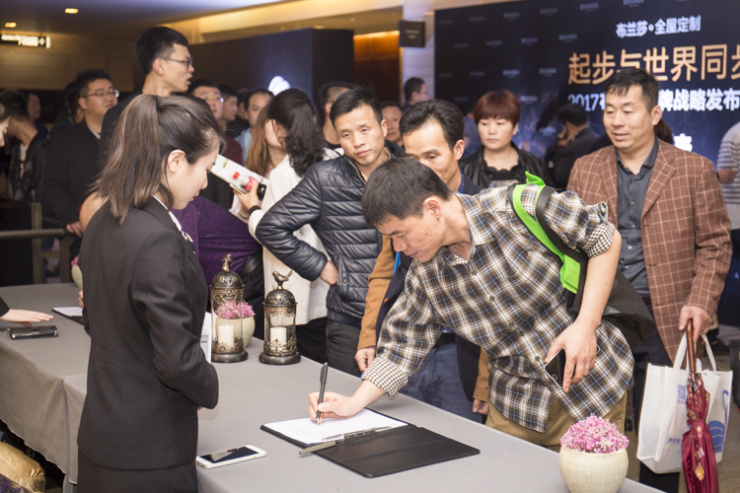发布会现场还有广东电视台、南方都市报、新快报、凤凰网、新浪家居、腾讯家居、网易家居、搜狐家居、泛家居圈、中华衣柜网、全球加盟网、今日头条、中国建材网、九正建材网、众家家居等几十家媒体共同见证。

现场沙画：演绎世界因梦想而伟大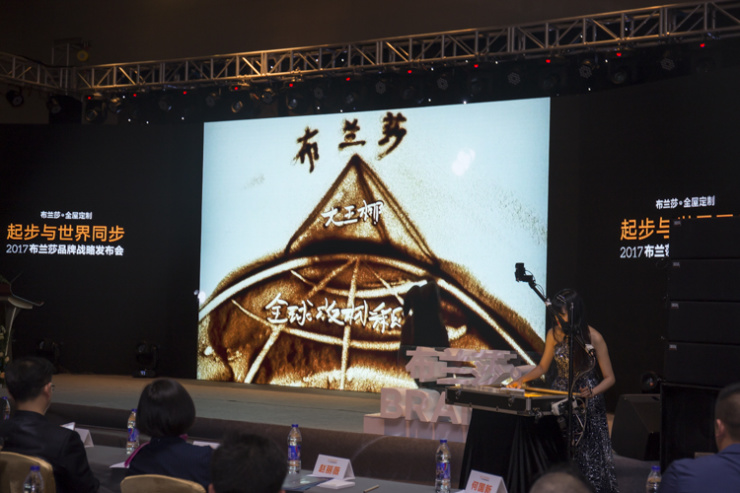布兰莎一出场就站在巨人的肩膀上，在定制家居的下半场，布兰莎将用资本力量重新定义游戏规则，必将成为定制家居领域内一颗耀眼的明星。下午3:30，品牌发布会在创意沙画表演中拉开帷幕，讲述从大王椰到布兰莎的17年梦想之路。大王椰用十七年做到板材翘楚，如今重新出发，进军定制行业。

曾秘书长：定制家居大未来 比拼的是资源整合能力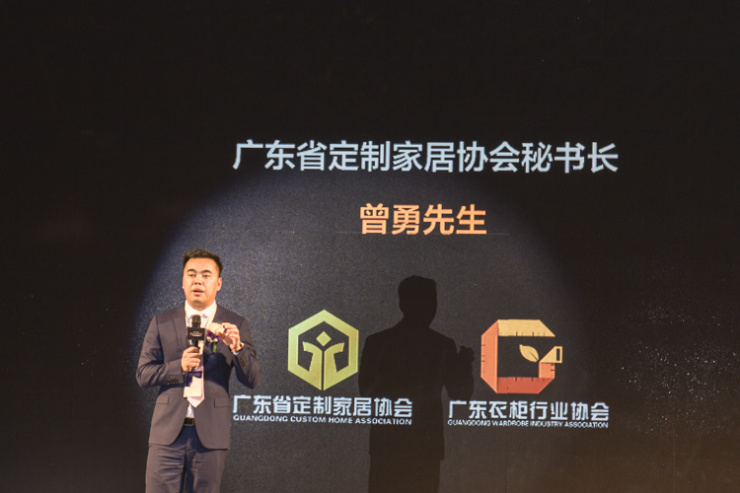品牌发布会首先由广东省定制家居协会秘书长曾勇先生上台致辞。曾秘书长提到：在大定制时代来临之际，消费趋势不断变化，而定制行业最核心的也是要不断满足消费者的消费趋势。2017年，为行业“定制融年”，具体为“资源融合”、“品类融合”、“区域融合”、“跨界融合”四个方面。企业之间比拼的是能够跟得上时代脚步的学习能力和资源整合能力。如今，跨界企业很多，但是很难成功，因为跨界的老板大多对自己的第二份事业没有了热情和专注。

但是布兰莎是个例外，大王椰二次创业的格局和魄力非常让人敬佩。顾国良董事长把布兰莎当成自己后半辈子的事业，正是因为顾董的专注和布兰莎团队的专注，相信布兰莎一定能够在定制家居行业走出新的高度。

顾国良董事长：创立布兰莎的使命与初心 现场公布六大战略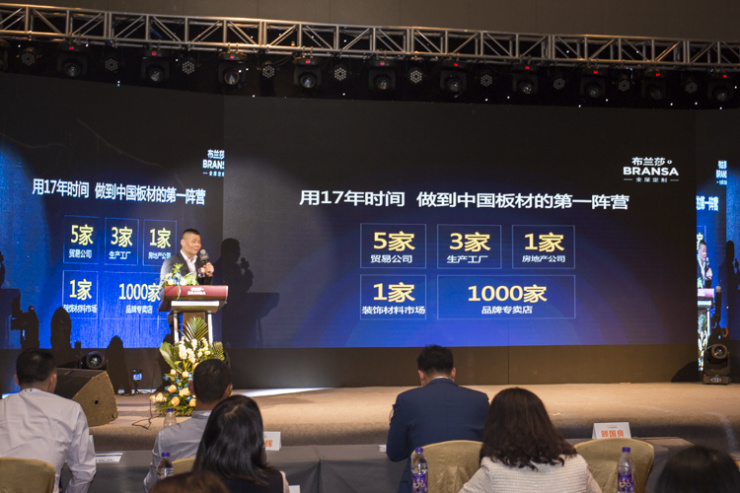业内外不少人有疑问：大王椰在板材行业做得风生水起，用17年做到板材的第一阵营，为什么要跨界？顾国良在现场给出了答案：为大王椰的命运而来，为颠覆行业格局而来。这样一个战略家的高瞻远瞩赢得了现场热烈的掌声。大王椰成功了，但是绝不止步于此，布兰莎迎着消费升级风口而来，感知着经销商之痛而来，为创造一个行业未来而来。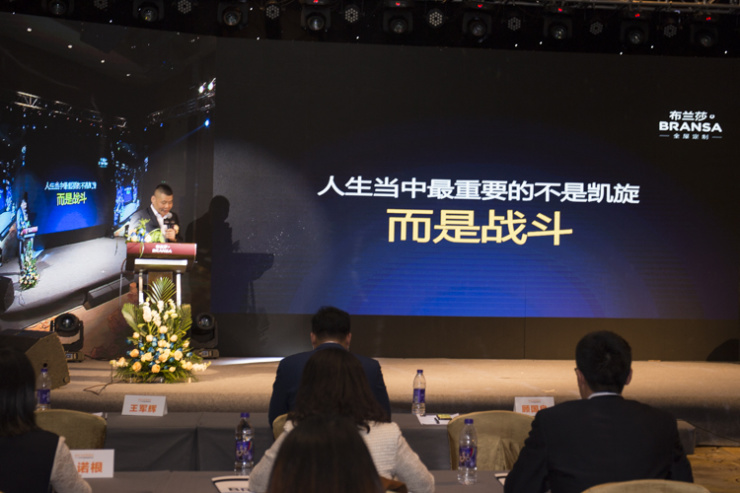同时，顾董还为布兰莎准备了一份大礼，那就是布兰莎“六大战略”，助力布兰莎飞速前行。最让人感动的就是顾董的“战略中的战略”：帮扶经销商。“用钱、用心、用团队”这句话，简简单单的7个字，让大家看到了一个企业家的魄力和决心，看到了一个追梦者的执着和激情，也看到了一个企业掌舵人对合作伙伴的真诚和用心——真正地将帮扶经销商作为布兰莎的第一使命。

营销副总李牛佳：深度解读“起步与世界同步”不是猛虎不下山布兰莎全屋定制此次发布会的主题是“起步与世界同步”，那么，这句话的具体内涵是是什么？布兰莎营销中心副总李牛佳在现场给予了精彩解读，那就是：“梦想与世界同步”、“智造与世界同步”、“设计与世界同步”、“服务与世界同步”、“团队与世界同步”。这就是布兰莎在定制家居行业下半场颠覆行业格局的底气所在。

李总提到：不是精英不聚首，不是猛虎不下山。现场布兰莎核心团队成员震撼亮相，他们真心实意地不惜一切代价助力布兰莎，帮扶经销商，服务客户。现场更有布兰莎的用户证言，布兰莎的产品和服务如何，他们最有发言权，在好口碑的背后是布兰莎的品质、服务、设计给予的信任，是布兰莎团队的同心协力。

市场中心副总高明明：布兰莎2017招商政策重磅发布定制家居谁最伤？还是经销商。布兰莎不仅拥有好的产品战略、好的团队、好的合作伙伴，最重要的是拥有一颗把经销商当家人的心。在发布会现场，市场中心副总高明明为大家带来了最有温度的招商政策，从展示设计到装修补贴、样品补贴、开业活动、培训、营运扶持、软件、安装、广告、返利，布兰莎10大帮扶体系，应该说是全方位、一条龙式地给予经销商无忧加盟。布兰莎让经销商一分投入，两分回报，与经销商组建命运共同体，打造利益共同体。

行业合作伙伴公约签署仪式 吹响集结的号角

布兰莎产品的品质能够始终如一，离不开顶尖的生产设备和软件支持，是国际领先的德国豪迈生产线让布兰莎实现揉性生产，产能翻番；是十万级的设计建模让布兰莎给予用户更多设计方案的选择，是合作伙伴的共同努力，才成就了布兰莎今天的起步与世界同步。会议现场，金田豪迈董事长关健华先生讲述了他和布兰莎之间的故事，持之以恒地将品质摆在第一位，是双方共同的目标，关董表示，希望在将来双方能够进一步深化合作，为布兰莎世界级的精工品质护航。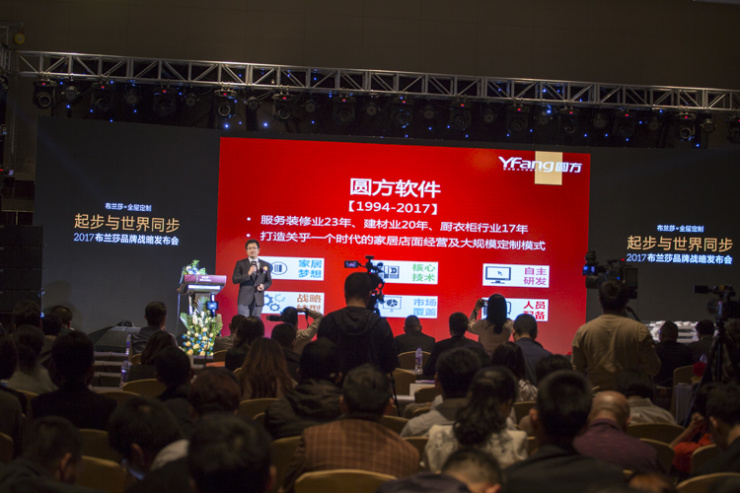此外，还有圆方软件副总经理丘权先生发言，让大家在现场真切地感受到了布兰莎在设计领域的独特竞争力，两位顶级合作伙伴均表示要更加深化与布兰莎的合作，给予布兰莎更加强大的市场竞争力。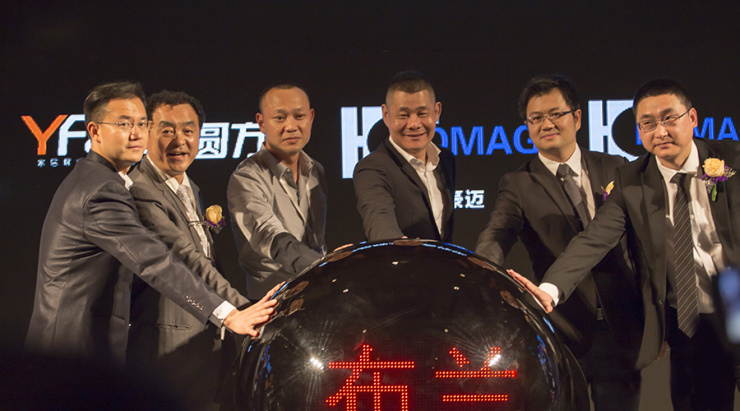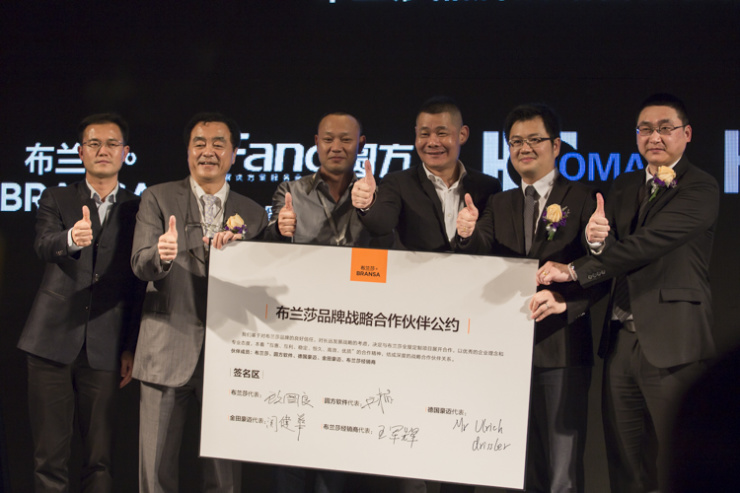最后，本次品牌发布会的核心亮点来袭：行业合作伙伴公约签署仪式。战略合作伙伴基于对布兰莎品牌的良好信任，对长远发展的战略考虑，与布兰莎全屋定制展开合作，以优秀的企业理念和专业态度，本着“互惠、互利、稳定、恒久、高效、优质”的合作精神，结成深度的战略合作伙伴关系。此份《伙伴公约》，致力于打造命运共同体，并共同为用户价值代言，促进行业健康发展。随后舞台上水晶球“布兰莎全屋定制——起步与世界同步”启动，宣告布兰莎时代来临了！此次品牌发布会在表演《布兰莎力量》中圆满落幕。此次广州震撼发声，布兰莎从此迈向了新的征程，迎来了品牌全新的时代，这是布兰莎发展史上又一个新的起点，一个新的里程碑，也必将成为中国定制家居行业领域内具有重大意义的事件。集结号已经吹响，梦想在这里起航，与布兰莎一起携手同行，一起开创定制家居的新时代。

`声明：本文由入驻焦点开放平台的作者撰写，除焦点官方账号外，观点仅代表作者本人，不代表焦点立场错误信息举报电话： 400-099-0099，邮箱：jubao@vip.sohu.com，或点此进行意见反馈，或点此进行举报投诉。`A B C D E F G H J K L M N P Q R S T W X Y Z
A - B - C - D - E
• A
• 鞍山
• 安庆
• 安阳
• 安顺
• 安康
• 澳门
• B
• 北京
• 保定
• 包头
• 巴彦淖尔
• 本溪
• 蚌埠
• 亳州
• 滨州
• 北海
• 百色
• 巴中
• 毕节
• 保山
• 宝鸡
• 白银
• 巴州
• C
• 承德
• 沧州
• 长治
• 赤峰
• 朝阳
• 长春
• 常州
• 滁州
• 池州
• 长沙
• 常德
• 郴州
• 潮州
• 崇左
• 重庆
• 成都
• 楚雄
• 昌都
• 慈溪
• 常熟
• D
• 大同
• 大连
• 丹东
• 大庆
• 东营
• 德州
• 东莞
• 德阳
• 达州
• 大理
• 德宏
• 定西
• 儋州
• 东平
• E
• 鄂尔多斯
• 鄂州
• 恩施
F - G - H - I - J
• F
• 抚顺
• 阜新
• 阜阳
• 福州
• 抚州
• 佛山
• 防城港
• G
• 赣州
• 广州
• 桂林
• 贵港
• 广元
• 广安
• 贵阳
• 固原
• H
• 邯郸
• 衡水
• 呼和浩特
• 呼伦贝尔
• 葫芦岛
• 哈尔滨
• 黑河
• 淮安
• 杭州
• 湖州
• 合肥
• 淮南
• 淮北
• 黄山
• 菏泽
• 鹤壁
• 黄石
• 黄冈
• 衡阳
• 怀化
• 惠州
• 河源
• 贺州
• 河池
• 海口
• 红河
• 汉中
• 海东
• 怀来
• I
• J
• 晋中
• 锦州
• 吉林
• 鸡西
• 佳木斯
• 嘉兴
• 金华
• 景德镇
• 九江
• 吉安
• 济南
• 济宁
• 焦作
• 荆门
• 荆州
• 江门
• 揭阳
• 金昌
• 酒泉
• 嘉峪关
K - L - M - N - P
• K
• 开封
• 昆明
• 昆山
• L
• 廊坊
• 临汾
• 辽阳
• 连云港
• 丽水
• 六安
• 龙岩
• 莱芜
• 临沂
• 聊城
• 洛阳
• 漯河
• 娄底
• 柳州
• 来宾
• 泸州
• 乐山
• 六盘水
• 丽江
• 临沧
• 拉萨
• 林芝
• 兰州
• 陇南
• M
• 牡丹江
• 马鞍山
• 茂名
• 梅州
• 绵阳
• 眉山
• N
• 南京
• 南通
• 宁波
• 南平
• 宁德
• 南昌
• 南阳
• 南宁
• 内江
• 南充
• P
• 盘锦
• 莆田
• 平顶山
• 濮阳
• 攀枝花
• 普洱
• 平凉
Q - R - S - T - W
• Q
• 秦皇岛
• 齐齐哈尔
• 衢州
• 泉州
• 青岛
• 清远
• 钦州
• 黔南
• 曲靖
• 庆阳
• R
• 日照
• 日喀则
• S
• 石家庄
• 沈阳
• 双鸭山
• 绥化
• 上海
• 苏州
• 宿迁
• 绍兴
• 宿州
• 三明
• 上饶
• 三门峡
• 商丘
• 十堰
• 随州
• 邵阳
• 韶关
• 深圳
• 汕头
• 汕尾
• 三亚
• 三沙
• 遂宁
• 山南
• 商洛
• 石嘴山
• T
• 天津
• 唐山
• 太原
• 通辽
• 铁岭
• 泰州
• 台州
• 铜陵
• 泰安
• 铜仁
• 铜川
• 天水
• 天门
• W
• 乌海
• 乌兰察布
• 无锡
• 温州
• 芜湖
• 潍坊
• 威海
• 武汉
• 梧州
• 渭南
• 武威
• 吴忠
• 乌鲁木齐
X - Y - Z
• X
• 邢台
• 徐州
• 宣城
• 厦门
• 新乡
• 许昌
• 信阳
• 襄阳
• 孝感
• 咸宁
• 湘潭
• 湘西
• 西双版纳
• 西安
• 咸阳
• 西宁
• 仙桃
• 西昌
• Y
• 运城
• 营口
• 盐城
• 扬州
• 鹰潭
• 宜春
• 烟台
• 宜昌
• 岳阳
• 益阳
• 永州
• 阳江
• 云浮
• 玉林
• 宜宾
• 雅安
• 玉溪
• 延安
• 榆林
• 银川
• Z
• 张家口
• 镇江
• 舟山
• 漳州
• 淄博
• 枣庄
• 郑州
• 周口
• 驻马店
• 株洲
• 张家界
• 珠海
• 湛江
• 肇庆
• 中山
• 自贡
• 资阳
• 遵义
• 昭通
• 张掖
• 中卫

1室1厅1厨1卫1阳台

1
2
3
4
5

0
1
2

1

1

0
1
2
3报名成功，资料已提交审核A B C D E F G H J K L M N P Q R S T W X Y Z
A - B - C - D - E
• A
• 鞍山
• 安庆
• 安阳
• 安顺
• 安康
• 澳门
• B
• 北京
• 保定
• 包头
• 巴彦淖尔
• 本溪
• 蚌埠
• 亳州
• 滨州
• 北海
• 百色
• 巴中
• 毕节
• 保山
• 宝鸡
• 白银
• 巴州
• C
• 承德
• 沧州
• 长治
• 赤峰
• 朝阳
• 长春
• 常州
• 滁州
• 池州
• 长沙
• 常德
• 郴州
• 潮州
• 崇左
• 重庆
• 成都
• 楚雄
• 昌都
• 慈溪
• 常熟
• D
• 大同
• 大连
• 丹东
• 大庆
• 东营
• 德州
• 东莞
• 德阳
• 达州
• 大理
• 德宏
• 定西
• 儋州
• 东平
• E
• 鄂尔多斯
• 鄂州
• 恩施
F - G - H - I - J
• F
• 抚顺
• 阜新
• 阜阳
• 福州
• 抚州
• 佛山
• 防城港
• G
• 赣州
• 广州
• 桂林
• 贵港
• 广元
• 广安
• 贵阳
• 固原
• H
• 邯郸
• 衡水
• 呼和浩特
• 呼伦贝尔
• 葫芦岛
• 哈尔滨
• 黑河
• 淮安
• 杭州
• 湖州
• 合肥
• 淮南
• 淮北
• 黄山
• 菏泽
• 鹤壁
• 黄石
• 黄冈
• 衡阳
• 怀化
• 惠州
• 河源
• 贺州
• 河池
• 海口
• 红河
• 汉中
• 海东
• 怀来
• I
• J
• 晋中
• 锦州
• 吉林
• 鸡西
• 佳木斯
• 嘉兴
• 金华
• 景德镇
• 九江
• 吉安
• 济南
• 济宁
• 焦作
• 荆门
• 荆州
• 江门
• 揭阳
• 金昌
• 酒泉
• 嘉峪关
K - L - M - N - P
• K
• 开封
• 昆明
• 昆山
• L
• 廊坊
• 临汾
• 辽阳
• 连云港
• 丽水
• 六安
• 龙岩
• 莱芜
• 临沂
• 聊城
• 洛阳
• 漯河
• 娄底
• 柳州
• 来宾
• 泸州
• 乐山
• 六盘水
• 丽江
• 临沧
• 拉萨
• 林芝
• 兰州
• 陇南
• M
• 牡丹江
• 马鞍山
• 茂名
• 梅州
• 绵阳
• 眉山
• N
• 南京
• 南通
• 宁波
• 南平
• 宁德
• 南昌
• 南阳
• 南宁
• 内江
• 南充
• P
• 盘锦
• 莆田
• 平顶山
• 濮阳
• 攀枝花
• 普洱
• 平凉
Q - R - S - T - W
• Q
• 秦皇岛
• 齐齐哈尔
• 衢州
• 泉州
• 青岛
• 清远
• 钦州
• 黔南
• 曲靖
• 庆阳
• R
• 日照
• 日喀则
• S
• 石家庄
• 沈阳
• 双鸭山
• 绥化
• 上海
• 苏州
• 宿迁
• 绍兴
• 宿州
• 三明
• 上饶
• 三门峡
• 商丘
• 十堰
• 随州
• 邵阳
• 韶关
• 深圳
• 汕头
• 汕尾
• 三亚
• 三沙
• 遂宁
• 山南
• 商洛
• 石嘴山
• T
• 天津
• 唐山
• 太原
• 通辽
• 铁岭
• 泰州
• 台州
• 铜陵
• 泰安
• 铜仁
• 铜川
• 天水
• 天门
• W
• 乌海
• 乌兰察布
• 无锡
• 温州
• 芜湖
• 潍坊
• 威海
• 武汉
• 梧州
• 渭南
• 武威
• 吴忠
• 乌鲁木齐
X - Y - Z
• X
• 邢台
• 徐州
• 宣城
• 厦门
• 新乡
• 许昌
• 信阳
• 襄阳
• 孝感
• 咸宁
• 湘潭
• 湘西
• 西双版纳
• 西安
• 咸阳
• 西宁
• 仙桃
• 西昌
• Y
• 运城
• 营口
• 盐城
• 扬州
• 鹰潭
• 宜春
• 烟台
• 宜昌
• 岳阳
• 益阳
• 永州
• 阳江
• 云浮
• 玉林
• 宜宾
• 雅安
• 玉溪
• 延安
• 榆林
• 银川
• Z
• 张家口
• 镇江
• 舟山
• 漳州
• 淄博
• 枣庄
• 郑州
• 周口
• 驻马店
• 株洲
• 张家界
• 珠海
• 湛江
• 肇庆
• 中山
• 自贡
• 资阳
• 遵义
• 昭通
• 张掖
• 中卫• 手机• 分享
• 设计
免费设计
• 计算器
装修计算器
• 入驻
合作入驻
• 联系
联系我们
• 置顶
返回顶部